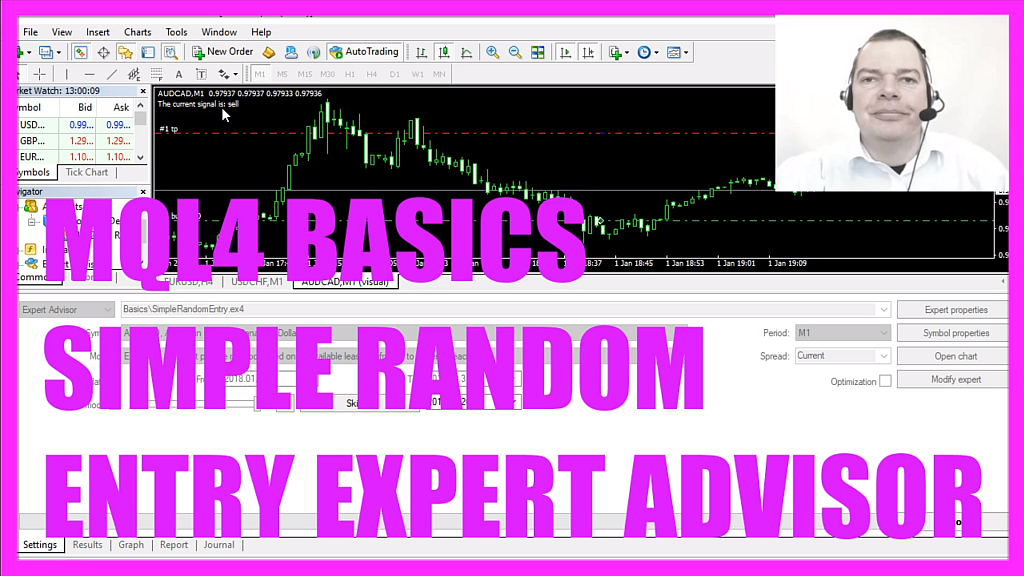In this video we are going to create random based entry signals, that might sound a little strange but I promise that the results will be very interesting, so let’s find out how to do that with mql4.
To get started please click on the little icon here or press F4 on your keyboard, now you should see the Metaeditor window and here you want to click on file, new file, expert advisor from template, continue, I will call this file simple random entry ea, click on continue, continue and finish.
Now you can delete everything above the on tick function and the two comment lines here, we start by creating an integer variable with the modifier extern; that will allow the user to change the setting for this variable and it will become interesting in the next video when we are going to create mass tests.
Inside of the on tick function, we start by creating a signal but we don’t assign any value here because we are going to calculate that now.
First, we start with this function, it is called math srand and when you mark it and press F1 you will learn that it sets the starting point for generating a series of pseudorandom integer numbers and to get a unique starting value we use get tick count as a parameter because get tick count returns the number of milliseconds that have passed since we have started our system and that number will always be unique and therefore the combination of these two functions will always create a unique series of random numbers.
To calculate our random number we use math rand, that’s a different one, this one is with an additional s and math rand returns pseudorandom integer within the range of zero to thirty-two thousand but with this expression, we will get two different values, either a zero or a one and if our random number equals zero we want to buy so we assign the value buy to our signal.
Otherwise, if the random number equals one, we want to sell so now we assign the value sell to our signal.
And if our signal equals buy and the value for orders total is zero – that would mean that we have no open orders and we have a buy signal – and now we use order send to buy ten micro lot.
Otherwise, if the signal equals sell and orders total is also zero – that would mean we have no open orders and a sell signal – and now we use order send to sell ten micro lot.
Finally, we use the comment function to output the text the current signal is followed by the calculated signal.